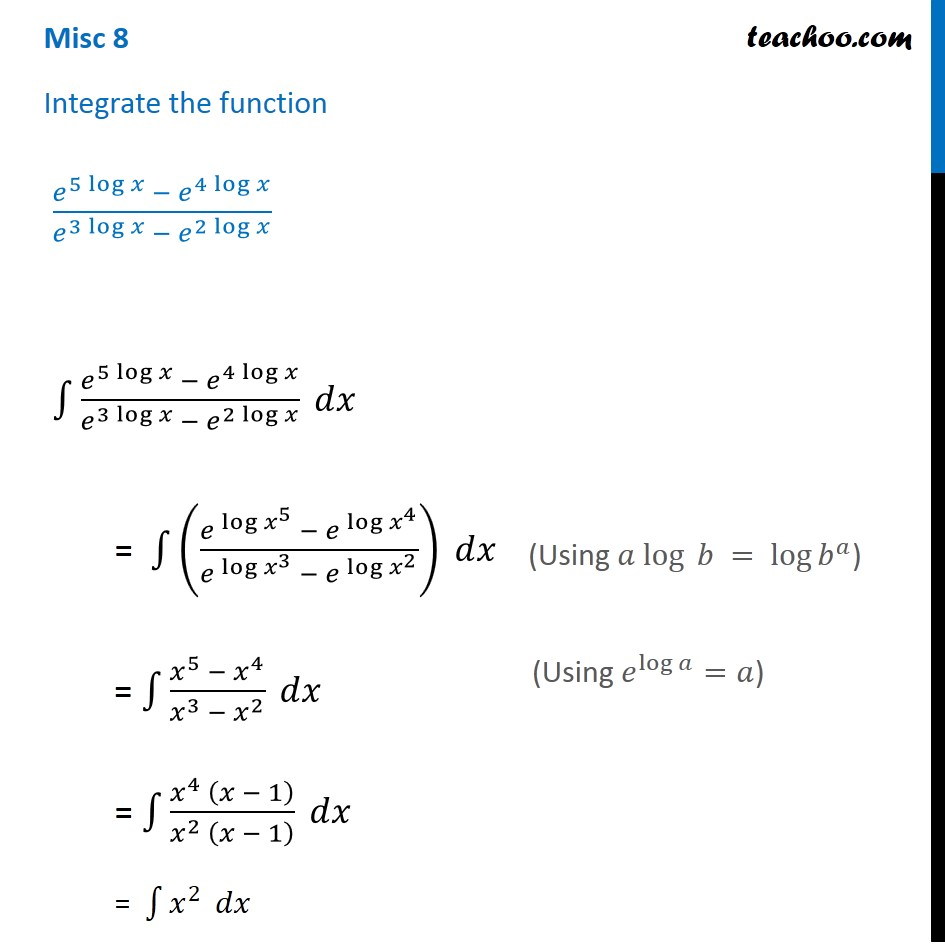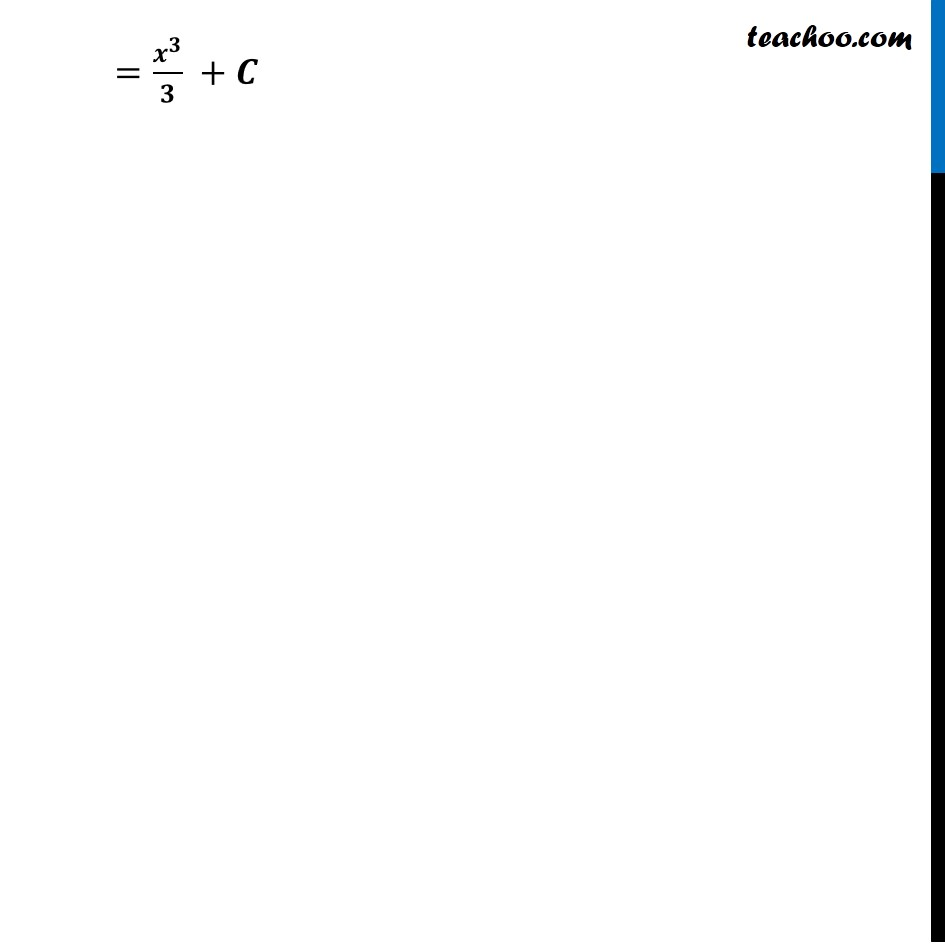Miscellaneous

Chapter 7 Class 12 Integrals
Serial order wiseLearn in your speed, with individual attention - Teachoo Maths 1-on-1 Class

### Transcript

Misc 8 Integrate the function (𝑒^(5 log⁡𝑥 ) − 𝑒^(4 log⁡𝑥 ))/(𝑒^(3 log⁡𝑥 ) − 𝑒^(2 log⁡𝑥 ) ) ∫1▒(𝑒^(5 log⁡𝑥 ) − 𝑒^(4 log⁡𝑥 ))/(𝑒^(3 log⁡𝑥 ) − 𝑒^(2 log⁡𝑥 ) ) 𝑑𝑥 = ∫1▒((〖𝑒 〗^log⁡〖𝑥^5 〗 − 𝑒^( log⁡〖𝑥^4 〗 ))/(𝑒^( log⁡〖𝑥^3 〗 ) − 𝑒^( log⁡〖𝑥^2 〗 ) )) 𝑑𝑥 = ∫1▒(𝑥^5 − 𝑥^4)/(𝑥^3 − 𝑥^2 ) 𝑑𝑥 = ∫1▒(𝑥^4 (𝑥 − 1))/(𝑥^2 (𝑥 − 1) ) 𝑑𝑥 = ∫1▒𝑥^2 𝑑𝑥 =𝒙^𝟑/𝟑 +𝑪 (Using 𝑎 log⁡ 𝑏 = log⁡𝑏^𝑎) (Using 𝑒^(log 𝑎)=𝑎) =𝒙^𝟑/𝟑 +𝑪